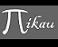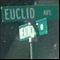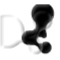Discussion about math, puzzles, games and fun.   Useful symbols: ÷ × ½ √ ∞ ≠ ≤ ≥ ≈ ⇒ ± ∈ Δ θ ∴ ∑ ∫  π  -¹ ² ³ °

You are not logged in.

## #1 2007-12-11 06:10:18

mikau
MemberRegistered: 2005-08-22
Posts: 1,504

### super fast trig functions

I was just thinking of some ways you could compute maclaurin or taylor expansions as quick as possible. Consider the maclauren expansion for sin(x)

or in sumation:

note that

(2n -1)! = (2n-1)(2n-2)(2n - 3)! and (2n - 3)! is the factorial of the preceding term.

Also

which also appears in the preceding term.  Thus

or if we allow n to move up in increments of 2 and shift the starting point, then we have

thus we could compute the sum as follows:

``````double xSquared = x*x; // compute x squared ahead of time

double sum = x; // add the first term to the accumulated sum
double previousTerm = x;
double currentTerm;

for (int n = 3; n < INFINITY; n+= 2) // continue on from the second term
{
// compute this term using the previous term
currentTerm = -previousTerm*xSquared/( (n*(n-1));
sum += currenTerm;
previousTerm = currentTerm;
}

return sum;``````

what do you think? Any ways we could make it even faster?

Last edited by mikau (2007-12-11 06:32:59)

A logarithm is just a misspelled algorithm.

Offline

## #2 2007-12-12 04:20:50

PHP Genius
Member
Registered: 2007-12-04
Posts: 5

### Re: super fast trig functions

I'm not very good with C/C++ (I think it's one of those) but wouldn't this:

``````double xSquared = x*x; // compute x squared ahead of time

double sum = x; // add the first term to the accumulated sum
double previousTerm = x;

for (int n = 3; n < INFINITY; n+= 2) // continue on from the second term
{
// compute this term using the previous term
previousTerm = -previousTerm*xSquared/( (n*(n-1));
sum += previousTerm;
}

return sum;``````

Be a little quicker?

Offline

## #3 2007-12-12 04:52:39

mikau
MemberRegistered: 2005-08-22
Posts: 1,504

### Re: super fast trig functions

you're right! thats a wasted statement.

Perhaps now I should consider how many iterations are necessary based on the input size.

A logarithm is just a misspelled algorithm.

Offline

## #4 2007-12-12 05:28:01

PHP Genius
Member
Registered: 2007-12-04
Posts: 5

### Re: super fast trig functions

This is only small but I think computers execute ((n^2)-n) quicker than (n*(n-1)). As I said in the last post though I'm not very good in C/C++ so this could be wrong. It might not be worth the hassle of changing and testing as it would only be a very very small gain in speed, if any.

Offline

## #5 2007-12-12 05:32:29

Ricky
ModeratorRegistered: 2005-12-04
Posts: 3,791

### Re: super fast trig functions

Playing around with optimizing code, and reinventing the wheel is never a bad idea when it comes to academic purposes.  But...

...keep in mind that you won't even get close to the library trig functions.  Hash tables don't even beat them:

``````#include <cstdlib>
#include <iostream>
#include <cmath>
#include <ctime>
#include <vector>

using namespace std;

vector<double> gerenateSineValues(int decimals) {
vector<double> result;
double d = pow((double)10, decimals);
int i;
for (i = 0; i < pow((double)10, decimals+1); i++) {
result.push_back(sin(i/d));
}
return result;
}

int main ()
{
time_t start_hash, end_hash, start_lib, end_lib;
int n, r, loops = 100000000;
double r2;
vector<double> sinValues = gerenateSineValues(5);
srand(time(NULL));

cout << "Starting hash method...";
cout.flush();
start_hash = clock();
for (n = 0; n < loops; n++) {
r = rand() % 1000000; //5 decimal places, 1 integer
sinValues[r];
}
end_hash = clock();
cout << "DONE" << endl;

cout << "Starting lib method...";
cout.flush();
start_lib = clock();
for (n = 0; n < loops; n++) {
r2 = (double)(rand() % 10000000)/((double)RAND_MAX+1);
sin(r2);
}
end_lib = clock();
cout << "DONE" << endl;

cout << "Hash: " << end_hash - start_hash << endl;
cout << "Lib: " << end_lib - start_lib << endl;

cout << endl;

return 0;
}``````

Output:

``````[root@localhost Desktop]# ./a.out
Starting hash method...DONE
Starting lib method...DONE
Hash: 7850000
Lib: 5320000``````

"In the real world, this would be a problem.  But in mathematics, we can just define a place where this problem doesn't exist.  So we'll go ahead and do that now..."

Offline

## #6 2007-12-12 07:48:11

mikau
MemberRegistered: 2005-08-22
Posts: 1,504

### Re: super fast trig functions

sheesh! why are the library functions so fast? Do they use... magic?

A logarithm is just a misspelled algorithm.

Offline

## #7 2007-12-12 08:01:06

PHP Genius
Member
Registered: 2007-12-04
Posts: 5

### Re: super fast trig functions

I think in most computers they have tables like how ricky did it. The only difference being they can access the table quicker as it's part of the CPU, not stored in memory.

Offline

## #8 2007-12-12 08:29:10

luca-deltodesco
MemberRegistered: 2006-05-05
Posts: 1,470

### Re: super fast trig functions

im not sure, but i believe that functions like sine and cosine are hardware implemented.

The Beginning Of All Things To End.
The End Of All Things To Come.

Offline

## #9 2007-12-12 12:07:13

Ricky
ModeratorRegistered: 2005-12-04
Posts: 3,791

### Re: super fast trig functions

Luca and PHPG have it right.  But I discovered something interesting when I wrote my code.  Sin function does take longer for very high values.  For example, sin(100000).  Anyone want to try to see what the big O is for the algorithm the library uses to reduce x into the 0 to 2pi range?

"In the real world, this would be a problem.  But in mathematics, we can just define a place where this problem doesn't exist.  So we'll go ahead and do that now..."

Offline

## #10 2007-12-13 13:05:27

mikau
MemberRegistered: 2005-08-22
Posts: 1,504

### Re: super fast trig functions

well how do we measure the input size?  just in flat radians?

I'll try testing in both java and C++, and let you know what I find.

A logarithm is just a misspelled algorithm.

Offline

## #11 2007-12-13 18:19:22

mikau
MemberRegistered: 2005-08-22
Posts: 1,504

### Re: super fast trig functions

well i just tried testing it in java, however i had to start and stop the watch outside a for loop because the functions were too fast for the difference to turn out greater than zero. Anway, here's the code:

``````
int loops = 1000000;

long startTime, stopTime, elapsedTime;

for (int inputSize = 0; inputSize <= 3200000; inputSize += 100000)
{
startTime = System.currentTimeMillis();
for (int i = 0; i < loops; i++)
{

Math.sin(inputSize);

}
stopTime = System.currentTimeMillis();

elapsedTime = stopTime - startTime;

System.out.println("input size: " + inputSize + " elapsed Time: " + elapsedTime );

}

}``````

output:

``````input size: 0 elapsed Time: 109
input size: 100000 elapsed Time: 437
input size: 200000 elapsed Time: 422
input size: 300000 elapsed Time: 422
input size: 400000 elapsed Time: 437
input size: 500000 elapsed Time: 438
input size: 600000 elapsed Time: 469
input size: 700000 elapsed Time: 421
input size: 800000 elapsed Time: 438
input size: 900000 elapsed Time: 437
input size: 1000000 elapsed Time: 454
input size: 1100000 elapsed Time: 406
input size: 1200000 elapsed Time: 437
input size: 1300000 elapsed Time: 422
input size: 1400000 elapsed Time: 438
input size: 1500000 elapsed Time: 422
input size: 1600000 elapsed Time: 421
input size: 1700000 elapsed Time: 2922
input size: 1800000 elapsed Time: 3016
input size: 1900000 elapsed Time: 2953
input size: 2000000 elapsed Time: 2843
input size: 2100000 elapsed Time: 2969
input size: 2200000 elapsed Time: 2625
input size: 2300000 elapsed Time: 2781
input size: 2400000 elapsed Time: 2719
input size: 2500000 elapsed Time: 2719
input size: 2600000 elapsed Time: 2906
input size: 2700000 elapsed Time: 2797
input size: 2800000 elapsed Time: 2797
input size: 2900000 elapsed Time: 2735
input size: 3000000 elapsed Time: 2750
input size: 3100000 elapsed Time: 2703
input size: 3200000 elapsed Time: 3047
Press any key to continue . . .``````

i tested it several times and it consistently jumped to a much longer time after about the 1.6 million mark. My guess is the algorithm checks to see if the input is bigger than something around 1.6 million and if so, does things differently.

A logarithm is just a misspelled algorithm.

Offline

## #12 2007-12-14 10:50:37

Ricky
ModeratorRegistered: 2005-12-04
Posts: 3,791

### Re: super fast trig functions

Quite an interesting find.  Once I get the time I'll play with it a bit myself.

"In the real world, this would be a problem.  But in mathematics, we can just define a place where this problem doesn't exist.  So we'll go ahead and do that now..."

Offline

## #13 2007-12-15 11:39:23

Ricky
ModeratorRegistered: 2005-12-04
Posts: 3,791

### Re: super fast trig functions

I found a much more sinusoidal pattern.  However, this could be due to processes in the background.  I'm going to do a more extensive test over night to see if it is.

``````Sin of 2480000 took 500000
Sin of 2490000 took 490000
Sin of 2500000 took 480000
Sin of 2510000 took 830000
Sin of 2520000 took 850000
Sin of 2530000 took 830000
Sin of 2540000 took 830000
Sin of 2550000 took 830000
Sin of 2560000 took 490000
Sin of 2570000 took 500000
Sin of 2580000 took 500000
Sin of 2590000 took 490000
Sin of 2600000 took 480000
Sin of 2610000 took 850000
Sin of 2620000 took 830000
Sin of 2630000 took 860000
Sin of 2640000 took 820000
Sin of 2650000 took 820000
Sin of 2660000 took 500000
Sin of 2670000 took 490000
Sin of 2680000 took 510000
Sin of 2690000 took 490000
Sin of 2700000 took 490000
Sin of 2710000 took 830000
Sin of 2720000 took 830000
Sin of 2730000 took 850000
Sin of 2740000 took 830000
Sin of 2750000 took 830000
Sin of 2760000 took 500000
Sin of 2770000 took 490000
Sin of 2780000 took 510000
Sin of 2790000 took 480000
Sin of 2800000 took 490000
Sin of 2810000 took 840000
Sin of 2820000 took 850000
Sin of 2830000 took 850000``````
``````#include <cstdlib>
#include <iostream>
#include <cmath>
#include <ctime>
#include <vector>

using namespace std;

int main ()
{
time_t start, end;
int i, n = 10000000;
int sin_val;
int sin_max = 100000000;
int sin_step = 10000;

cout << "n: " << n << endl;

for (sin_val = 0; sin_val < 100000000; sin_val += sin_step) {
start = clock();
for (i = 0; i < n; i++) {
sin(sin_val);
}
end = clock();

cout << "Sin of " << sin_val << " took " << end - start << endl;
}

return 0;
}``````

"In the real world, this would be a problem.  But in mathematics, we can just define a place where this problem doesn't exist.  So we'll go ahead and do that now..."

Offline

## #14 2007-12-15 12:06:49

mikau
MemberRegistered: 2005-08-22
Posts: 1,504

### Re: super fast trig functions

hmmm... weird!

did you observe any jumps at some of the lower values as I did?

A logarithm is just a misspelled algorithm.

Offline

## #15 2007-12-15 12:53:58

Ricky
ModeratorRegistered: 2005-12-04
Posts: 3,791

### Re: super fast trig functions

All parts of the file look about the same.  I just posted a sample.

"In the real world, this would be a problem.  But in mathematics, we can just define a place where this problem doesn't exist.  So we'll go ahead and do that now..."

Offline